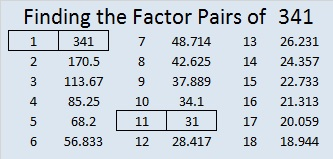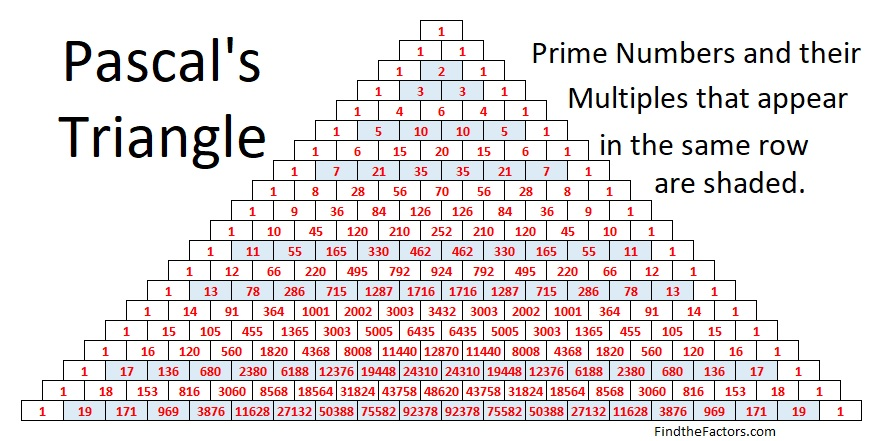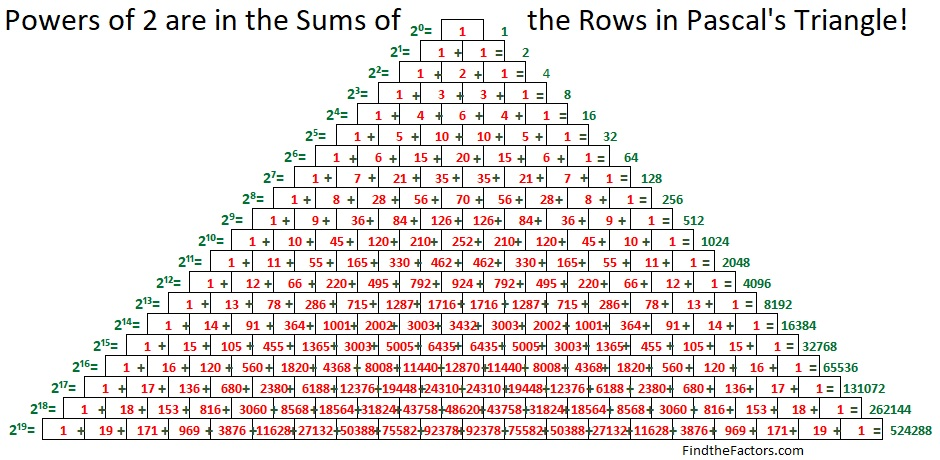# 341 is the smallest composite number that gives a false positive for this Quick Prime Number Test

• 341 is a composite number.
• Prime factorization: 341 = 11 x 31
• The exponents in the prime factorization are 1 and 1. Adding one to each and multiplying we get (1 + 1)(1 + 1) = 2 x 2 = 4. Therefore 341 has exactly 4 factors.
• Factors of 341: 1, 11, 31, 341
• Factor pairs: 341 = 1 x 341 or 11 x 31
• 341 has no square factors that allow its square root to be simplified. √341 ≈ 18.466341 is a composite number that sometimes acts like a prime number. To understand why, we need to understand a little bit about modular arithmetic:

When one number is divided by another, sometimes there is a remainder. Modular arithmetic is all about the remainders. We don’t care how many times one number divides into another number; we only care about the remainder.

Something very curious happens when the equation in the chart below is applied to a prime number greater than 2: The remainder is always 2. It really is! For example, 2^5 = 32 and 32 divided by 5 is 6R2. We say that 32 (mod 5) = 2 or 2^5 (mod 5) = 2 because 2 is the remainder. The fact that the remainder for prime numbers applied to this equation is always 2 is amazing, and can be a QUICK TEST to see if an odd number might be a PRIME NUMBER! If the remainder isn’t 2, that odd number is definitely NOT prime!QUICK PRIME NUMBER TEST (Please, excuse my using = instead of ≡, the “equal sign” that is usually used in modular arithmetic. I think = looks less intimidating.)

Passing the remainder test is a necessary but not a sufficient indicator that a number is prime: Even though 341 is not a prime number, the quantity 2^341 divided by 341 also has a remainder of 2. Since 341 = 11 x 31, but passes the remainder test, it is known as a pseudoprime number.  341 is the smallest composite number that passes this particular test, so 341 is an amazing number!

Also in the chart above, 2 is the most common remainder, followed by 8, then 32, then 128. All of those numbers are odd powers of 2. The even powers of 2 do not appear on the chart at all! That is a very curious phenomenon as well. (However, if x is an even number, it appears that y will usually be an even power of 2.)

Earlier mathematicians have written equivalent expressions and algorithms, but I prefer using “2^x (mod x)” because it takes very few keystrokes to enter into a calculator before hitting the equal sign:

Look at the image below.  It demonstrates that in prime-numbered rows, the numbers in that row can be divided evenly by that prime number (not counting the 1’s at the beginning and ending of each row).• For a composite number, such as 15, at least one of the numbers in the row will NOT be divisible by that composite number. In row 15, 1 is the 0th term, 15 is the 1st term, 105 is the 2nd term, 455 is the 3rd term, and 1365 is the 4th term and so forth.
• Terms that are divisible by 15 are the 1st term (15), the 2nd term (105), the 4th term (1365), the 7th term (6435), the 8th term (6435), the 11th term (1365), and the 13th term (105). All of those term numbers, 1, 2, 4, 7, 8, 11, and 13, do NOT have factors in common with the number 15.
• The terms that are NOT divisible by 15 are the 3rd term (455), the 5th term (3003), the 6th term (5005), the 9th term (5005), the 10th term (3003), and the 12th term (455). All of those term numbers, 3, 5, 6, 9, 10, and 12, have at least one factor in common with the number 15.

If we could see the VERY large numbers for the 341st row, and if they weren’t expressed in Scientific Notation, we could note that the following terms would NOT be divisible by 341: terms numbered 11th, 22nd, 33rd, 44th, and so forth and the terms numbered 31st, 62nd, 93rd and so forth. However, if you add those terms together, that very large sum would be divisible by 341. That is so amazing, even though 341 is not prime!

This second image from Pascal’s Triangle demonstrates that the sum of the numbers in any row of Pascal’s triangle equals two raised to the second number in that row.
(There is no second number in the top row, so we could say that second number is zero, and 2º = 1.)These two images work together so that 2^p (mod p) will always be 2 for every prime number greater than 2 because every number in the prime-numbered rows can be evenly divided by that prime number. (Except the 1 at the beginning of the row and the 1 at the end of the row; Note 1 + 1 = 2)

Now that is why this amazing test for prime numbers works as well as it does while giving just a few false positives!

## 9 thoughts on “341 is the smallest composite number that gives a false positive for this Quick Prime Number Test”

1.Joseph Nebus

I didn’t know this about 341; it’s an interesting property to have.

2.nerdinthebrain

That was really interesting! Who knew 341 was so awesome??? 😀

3.Steve Morris

Spooky.

4.Paula Beardell Krieg

I am printing this up and heading for my armchair…

5.Travis McCracken

All prime candidates through 5461 that yield a false positive for the remainder test n|((2^n)-2):

{341, 1387, 1729, 2047, 2701, 2821, 3277, 4033, 4369, 4681, 5461}

6.Richard Miner

If you write the pseudoprime number test in Python, it becomes (2**N)%N==2. And since we are working with 2 as the base number, using the left shift operator instead of raising to a power is faster to perform, thus (2**N)%N==2 can be rewritten as (1<<N)%N==2, which will greatly speed up the computation.

7.Richard Miner

It appears that the formula doesn’t have to use powers of 2. If you use any other whole number it seems to work. Thus (3**N)%N==3, (7**N)%N==7, etc. Oddly using numbers other than 2 as the base produce a larger number of pseudoprimes. Given the previously mentioned advantage of using base 2, these other bases become (possibly) less interesting, but it is rather peculiar that they still seem to work.

This site uses Akismet to reduce spam. Learn how your comment data is processed.